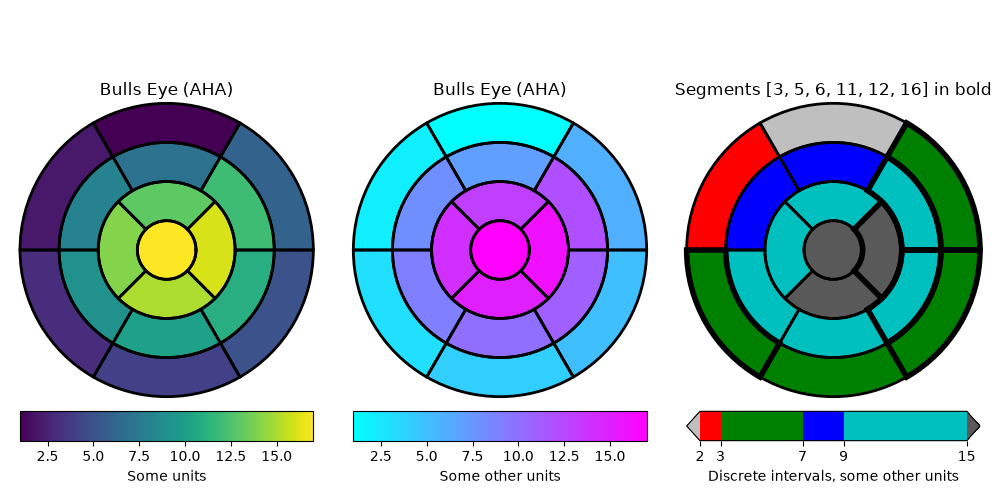# Left ventricle bullseye#

This example demonstrates how to create the 17 segment model for the left ventricle recommended by the American Heart Association (AHA).import matplotlib.pyplot as plt
import numpy as np

import matplotlib as mpl

def bullseye_plot(ax, data, seg_bold=None, cmap="viridis", norm=None):
"""
Bullseye representation for the left ventricle.

Parameters
----------
ax : axes
data : list[float]
The intensity values for each of the 17 segments.
seg_bold : list[int], optional
A list with the segments to highlight.
cmap : colormap, default: "viridis"
Colormap for the data.
norm : Normalize or None, optional
Normalizer for the data.

Notes
-----
This function creates the 17 segment model for the left ventricle according
to the American Heart Association (AHA) _

References
----------
..  M. D. Cerqueira, N. J. Weissman, V. Dilsizian, A. K. Jacobs,
S. Kaul, W. K. Laskey, D. J. Pennell, J. A. Rumberger, T. Ryan,
and M. S. Verani, "Standardized myocardial segmentation and
nomenclature for tomographic imaging of the heart",
Circulation, vol. 105, no. 4, pp. 539-542, 2002.
"""

data = np.ravel(data)
if seg_bold is None:
seg_bold = []
if norm is None:
norm = mpl.colors.Normalize(vmin=data.min(), vmax=data.max())

r = np.linspace(0.2, 1, 4)

ax.set(ylim=[0, 1], xticklabels=[], yticklabels=[])
ax.grid(False)  # Remove grid

# Fill segments 1-6, 7-12, 13-16.
for start, stop, r_in, r_out in [
(0, 6, r, r),
(6, 12, r, r),
(12, 16, r, r),
(16, 17, 0, r),
]:
n = stop - start
dtheta = 2*np.pi / n
ax.bar(np.arange(n) * dtheta + np.pi/2, r_out - r_in, dtheta, r_in,
color=cmap(norm(data[start:stop])))

# Now, draw the segment borders.  In order for the outer bold borders not
# to be covered by inner segments, the borders are all drawn separately
# after the segments have all been filled.  We also disable clipping, which
# would otherwise affect the outermost segment edges.
# Draw edges of segments 1-6, 7-12, 13-16.
for start, stop, r_in, r_out in [
(0, 6, r, r),
(6, 12, r, r),
(12, 16, r, r),
]:
n = stop - start
dtheta = 2*np.pi / n
ax.bar(np.arange(n) * dtheta + np.pi/2, r_out - r_in, dtheta, r_in,
clip_on=False, color="none", edgecolor="k", linewidth=[
4 if i + 1 in seg_bold else 2 for i in range(start, stop)])
# Draw edge of segment 17 -- here; the edge needs to be drawn differently,
# using plot().
ax.plot(np.linspace(0, 2*np.pi), np.linspace(r, r), "k",
linewidth=(4 if 17 in seg_bold else 2))

# Create the fake data
data = np.arange(17) + 1

# Make a figure and axes with dimensions as desired.
fig = plt.figure(figsize=(10, 5), layout="constrained")
axs = fig.subplots(1, 3, subplot_kw=dict(projection='polar'))
fig.canvas.manager.set_window_title('Left Ventricle Bulls Eyes (AHA)')

# Set the colormap and norm to correspond to the data for which
# the colorbar will be used.
cmap = mpl.cm.viridis
norm = mpl.colors.Normalize(vmin=1, vmax=17)
# Create an empty ScalarMappable to set the colorbar's colormap and norm.
# The following gives a basic continuous colorbar with ticks and labels.
fig.colorbar(mpl.cm.ScalarMappable(cmap=cmap, norm=norm),
cax=axs.inset_axes([0, -.15, 1, .1]),
orientation='horizontal', label='Some units')

# And again for the second colorbar.
cmap2 = mpl.cm.cool
norm2 = mpl.colors.Normalize(vmin=1, vmax=17)
fig.colorbar(mpl.cm.ScalarMappable(cmap=cmap2, norm=norm2),
cax=axs.inset_axes([0, -.15, 1, .1]),
orientation='horizontal', label='Some other units')

# The second example illustrates the use of a ListedColormap, a
# BoundaryNorm, and extended ends to show the "over" and "under"
# value colors.
cmap3 = (mpl.colors.ListedColormap(['r', 'g', 'b', 'c'])
.with_extremes(over='0.35', under='0.75'))
# If a ListedColormap is used, the length of the bounds array must be
# one greater than the length of the color list.  The bounds must be
# monotonically increasing.
bounds = [2, 3, 7, 9, 15]
norm3 = mpl.colors.BoundaryNorm(bounds, cmap3.N)
fig.colorbar(mpl.cm.ScalarMappable(cmap=cmap3, norm=norm3),
cax=axs.inset_axes([0, -.15, 1, .1]),
extend='both',
ticks=bounds,  # optional
spacing='proportional',
orientation='horizontal',
label='Discrete intervals, some other units')

# Create the 17 segment model
bullseye_plot(axs, data, cmap=cmap, norm=norm)
axs.set_title('Bulls Eye (AHA)')

bullseye_plot(axs, data, cmap=cmap2, norm=norm2)
axs.set_title('Bulls Eye (AHA)')

bullseye_plot(axs, data, seg_bold=[3, 5, 6, 11, 12, 16],
cmap=cmap3, norm=norm3)
axs.set_title('Segments [3, 5, 6, 11, 12, 16] in bold')

plt.show()


Total running time of the script: (0 minutes 1.018 seconds)

Gallery generated by Sphinx-Gallery